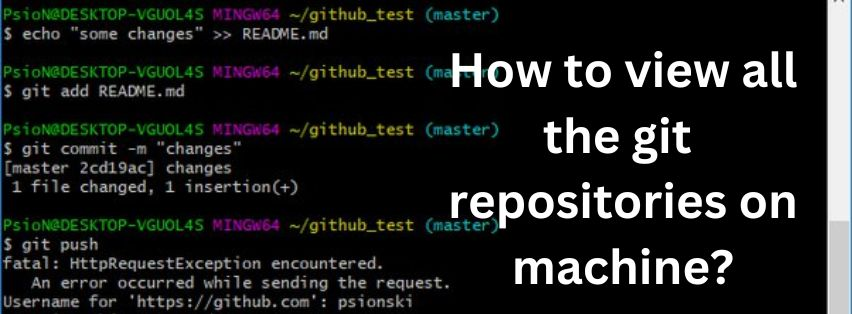Jully 18, 2022

### How to create lists from pandas columns?

Creating lists from pandas columns is a useful way to organize data for analysis. It can be used to create a variety of different types of lists, such as numeric, string, and boolean lists. In this essay, we will discuss the various ways to create lists from pandas columns, including the use of the pandas library, the list comprehension method, and the map() function. We will also discuss how to manipulate the data in the lists once they have been created.

### Using the Pandas Library

The pandas library is a powerful tool for data analysis and manipulation. It provides a variety of functions and methods that can be used to create lists from pandas columns. The most common way to create a list from a pandas column is to use the tolist() method. This method takes a pandas column as an argument and returns a list of the values in that column. For example, if we have a pandas DataFrame with a column called “Name”, we can use the tolist() method to create a list of all the names in that column:

names_list = df[‘Name’].tolist()

The tolist() method can also be used to create lists from multiple columns. To do this, we can pass multiple columns as arguments to the tolist() method. For example, if we have a pandas DataFrame with two columns called “Name” and “Age”, we can use the tolist() method to create a list of tuples containing the name and age of each person:

name_age_list = df[[‘Name’, ‘Age’]].tolist()

### Using List Comprehension

Another way to create lists from pandas columns is to use list comprehension. List comprehension is a powerful tool for creating lists from existing data. It allows us to quickly and easily create lists from pandas columns without having to write a lot of code. For example, if we have a pandas DataFrame with a column called “Name”, we can use list comprehension to create a list of all the names in that column:

names_list = [name for name in df[‘Name’]]

We can also use list comprehension to create lists from multiple columns. To do this, we can pass multiple columns as arguments to the list comprehension expression. For example, if we have a pandas DataFrame with two columns called “Name” and “Age”, we can use list comprehension to create a list of tuples containing the name and age of each person:

name_age_list = [(name, age) for name, age in zip(df[‘Name’], df[‘Age’])]

### Using the Map() Function

The map() function is another way to create lists from pandas columns. The map() function takes a function as an argument and applies it to each element in an iterable. For example, if we have a pandas DataFrame with a column called “Name”, we can use the map() function to create a list of all the names in that column:

names_list = list(map(lambda x: x, df[‘Name’]))

We can also use the map() function to create lists from multiple columns. To do this, we can pass multiple columns as arguments to the map() function. For example, if we have a pandas DataFrame with two columns called “Name” and “Age”, we can use the map() function to create a list of tuples containing the name and age of each person:

name_age_list = list(map(lambda x, y: (x, y), df[‘Name’], df[‘Age’]))

### Manipulating Data in Lists

Once we have created our lists from pandas columns, we may want to manipulate the data in those lists. This can be done using various methods such as sorting, filtering, and transforming.For example, if we have a list of tuples containing the name and age of each person, we can use list comprehension to create a new list containing only the names of people over 18 years old:

over_18_names = [name for name, age in name_age_list if age >= 18]

### Conclusion:

In conclusion, creating lists from pandas columns is an important step in data analysis and manipulation. There are several ways to do this, including using the pandas library, list comprehension, and the map() function. Once the lists have been created, they can be manipulated using various methods such as sorting, filtering, and transforming. With these tools at our disposal, we can easily organize and analyze our data.

### Recent Posts### Online revenue system review

Jully 11, 2022### How to view all the git repositories on machine?

Jully 11, 2022Jully 11, 2022